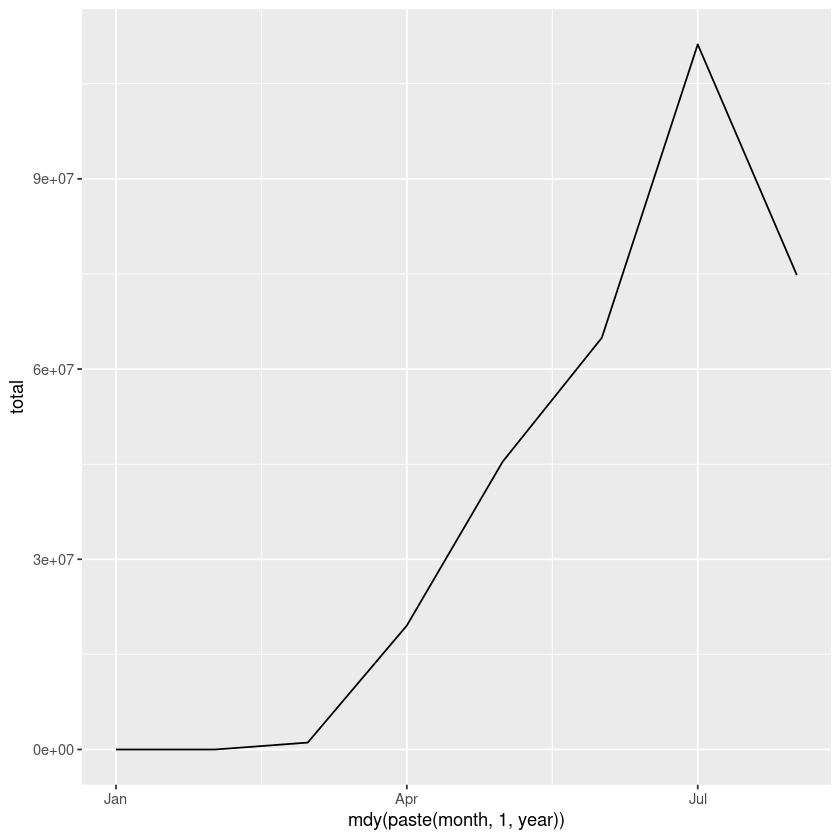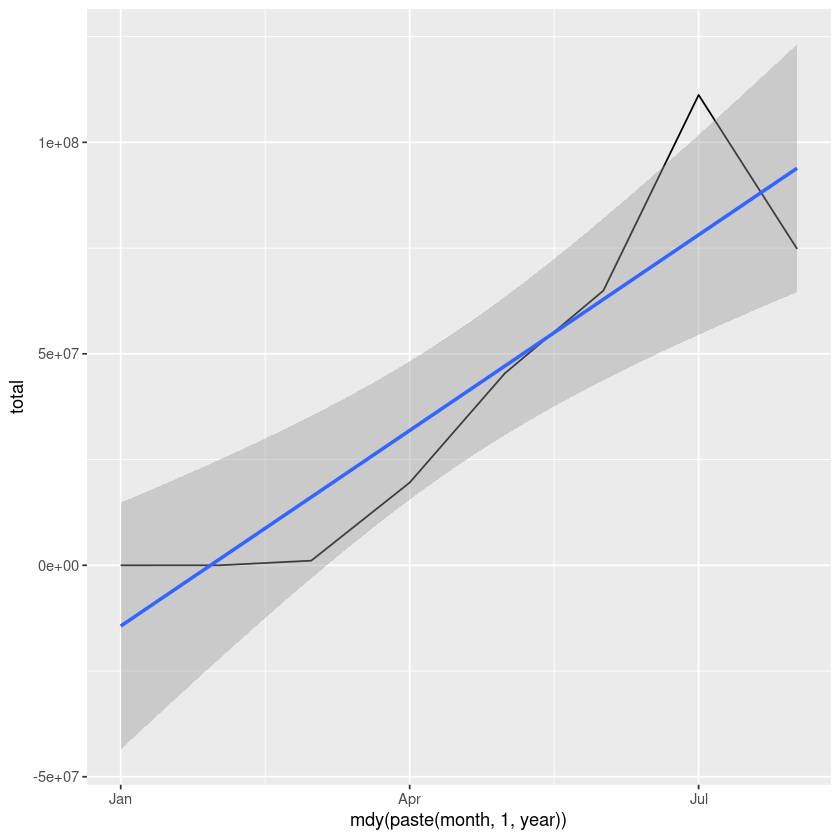# How To Add Regression Line On Ggplot

Let us import the neccessary packages first.

In :
library(ggplot2)
library(dplyr)
library(lubridate)


For the example data, we would analyze the covid19 data which is available on the github. We would do a line plot of monthly US data and then plot regression line on top of that plot.

In :
url <- "https://pomber.github.io/covid19/timeseries.json"
covid_data <- fromJSON(url,flatten = TRUE)


covid_data dataframe has data for all the countries, let us look at the data for US only.

In :
us_data <- covid_data$US  In : typeof(covid_data$US[0,'date'])

'character'

As we see above date is in character format. We need to change this format to date, since we want to group the data by month.

## Extract month and year from date column

In :
month = month(as.Date(covid_data$US[,'date'],format="%Y-%m-%d")) year = year(as.Date(covid_data$US[,'date'],format="%Y-%m-%d"))


Let us merge the month and year column to our dataframe.

In :
us_data <- mutate(us_data,month=month,year=year)


## Group data by month and year

We will use the dplyr package to summarize the data.

In :
us_data_gbymonth <- us_data %>% group_by(month,year) %>% summarize(total = sum(confirmed, na.rm = TRUE))

In :
us_data_gbymonth

A grouped_df: 8 × 3
monthyeartotal
<dbl><dbl><int>
12020 38
22020 378
32020 1091068
42020 19552582
52020 45407574
62020 64933835
72020111200861
82020 74821798

## Merging separate month and year columns to graph in ggplot2

We will use mdy function from lubridate package to join month and year columns as our x-axis.

In :
ggplot(us_data_gbymonth,aes(mdy(paste(month,1,year)),y=total)) + geom_line()## Add regression line on ggplot

Let add regression line on ggplot now. We would use stat_summary and geom_smoooth function.

In :
options(warn=-1)
ggplot(us_data_gbymonth,aes(x=mdy(paste(month,1,year)),y=total)) + geom_line() +
stat_summary(fun.data= mean_cl_normal) +  geom_smooth(method='lm')

geom_smooth() using formula 'y ~ x'